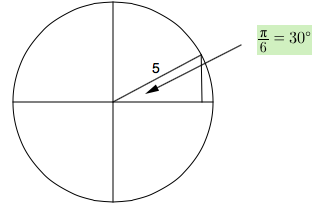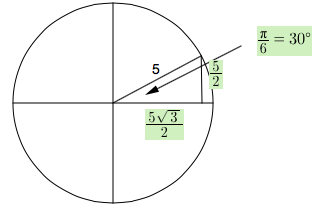### Home > PC3 > Chapter 7 > Lesson 7.2.1 > Problem7-76

7-76.

Given the magnitude and direction, express each vector in component form.

1. $\Vert\vec{\text{r}}\Vert=5$ and $θ=\frac{\pi}{6}$

Draw each vector in a circle. Then make a right triangle with the $x\text{-axis}$.

Circle with right triangle in first quadrant, hypotenuse labeled 5, central angle labeled, 1 sixth pi, = 30 degrees.

Solve the rest of the triangle.
Note: Each part forms a special right triangle.

Labels added, vertical leg, 5 halves, horizontal leg, 5 halves Times, Square root of 32. $\Vert\vec{\text{s}}\Vert=10$ and $θ=\frac{5\pi}{4}$

3. $\Vert\vec{\text{t}}\Vert=1$ and $θ=\frac{5\pi}{4}$